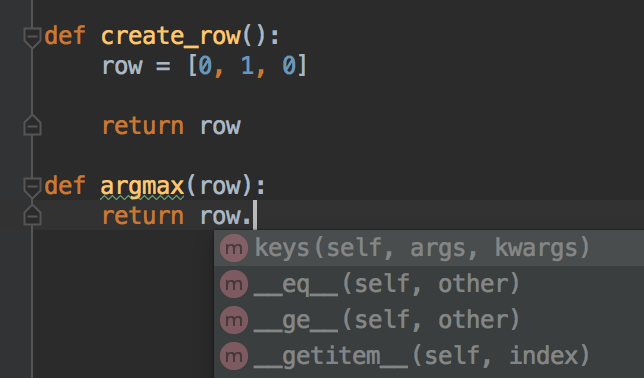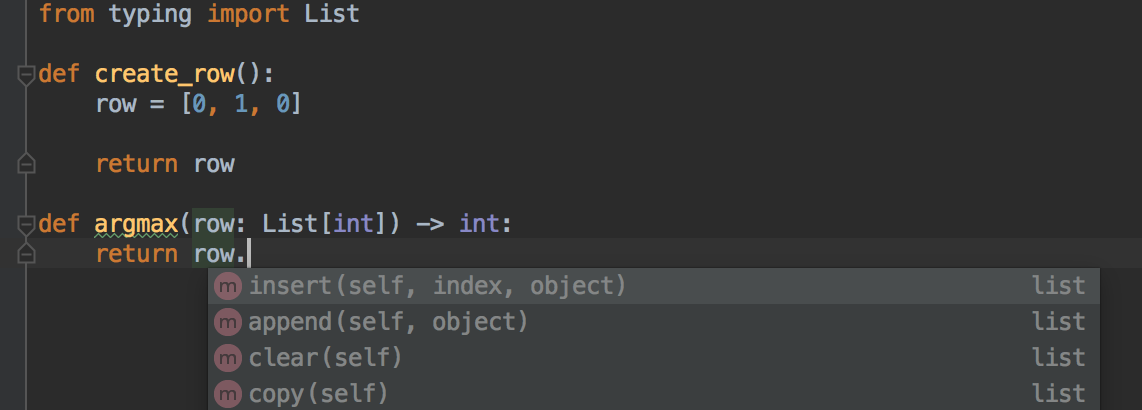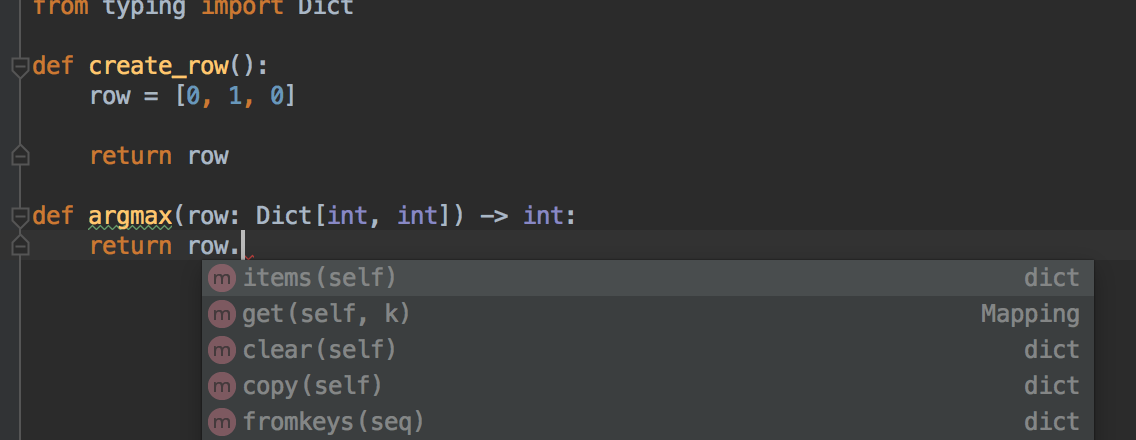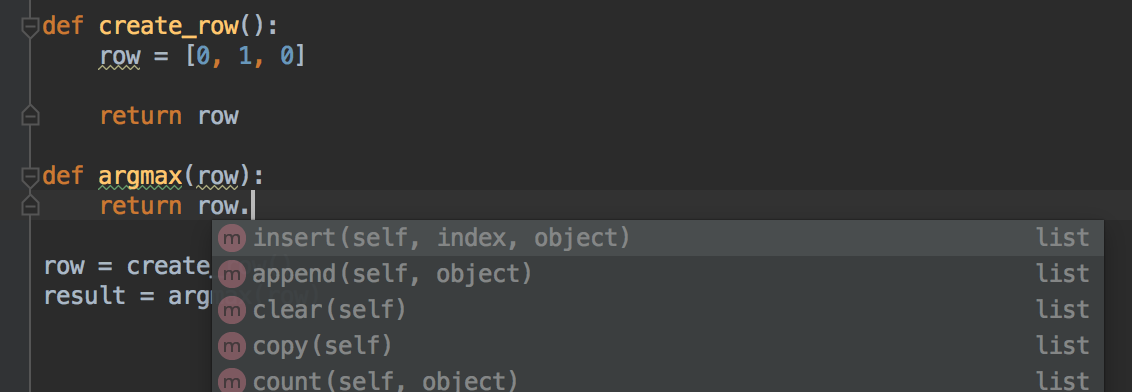# Static typing in Python

Posté le Sat 13 October 2018 dans coding • 5 min de lecture

Cet article est le numéro 2 de la série "type systems" :

In a previous blog post, I explained what static typing is, and presented a small example in Scala. We saw that static typing enables to spot some errors before executing the program. This is one of the reasons why Scala and Java are used in production for data pipelines. As Python is often used by data scientists to process data, early catching obviously (through type checking) erroneous code is beneficial for an industrialization of it, without rewriting it into another language.

Luckily, Python also has optional static typing since version 3.5. It enables types checking before execution with tools such as mypy, but also other powerfull features for IDEs as we will see in this blog post.

PEP 582 gives an overview for type hints before it was adopted by Python. The next section provides more references to type hints in Python, and presents some examples of the syntax.

## Type annotations in Python

PEP 483 introduced a discussion on type hints for Python. This PEP has the type "informational", meaning (see PEP 1) that it does not propose a new feature that should be implemented.

It is thus an introduction to PEP 484 which is in the "standards track", meaning it describes a new feature. The status is "provisional", i.e. the proposal has been accepted for inclusion in the reference implementation.

PEP 484 introduced both the typing module and a syntax for type annotations for function signatures.

Here is an instance of function annotation:

```def add_one(n: int) -> int:
return n + 1
```

The typing module provides additional types, for instance the type List:

```from typing import List

def append_one(l: List[int]) -> List[int]:
l += 1
```

It enables also type aliases:

```from typing import List

Vector = List[float]

def normalize(vector: Vector) -> Vector:
norm = sqrt(sum([e**2 for e in vector]))
return [e/norm for e in vector]
```

Here, we defined the Vector type as an alias for the type List[float].

PEP 484 also introduced a syntax for variable annotations with comments:

```integers = []  # type: List[int]
```

PEP 526 introduced another syntax for variable annotations without relying on comments to express the type of variables. For instance, PEP 526 allows the following syntax:

```integers: List[int] = []
```

For now, we only saw the basics of type annotations and their benefits from a documentation perspective: the documentation of the types of the inputs and outputs of functions, and the types of variables, is included into the code itself through type annotations. It is time to see them in action for static type checking and other features.

## Type checking with mypy

mypy is a static type checker for Python. Let's see a small example (example1.py):

```from typing import Dict

def create_row():
row = {'x': 0,
'y': 1,
'z': 0}

return row

def argmax(row: Dict[str, int]) -> str:
return max(row, key=row.get)

row = create_row()
result = argmax(row)
print(f"key: {result}, value: {row[result]}")
```

We created a row implemented by a dictionary where the keys are the column labels. We then print the maximum value of the row and its corresponding column.

We can check the types with mypy:

```mypy example1.py
```

It outputs nothing, meaning that nothing went wrong with the type checking.

Say we want to modify the implementation of a row by representing it as a list since every row values correspond to the same columns, but we forget to change the implementation of argmax:

```from typing import List, Dict

def create_row() -> List[int]:
row = [0, 1, 0]

return row

def argmax(row: Dict[str, int]) -> str:
return max(row, key=row.get)

row = create_row()
result = argmax(row)
print(f"key: {result}, value: {row[result]}")
```

If we run mypy on this example (example2.py), it outputs:

```example2.py:14: error: Argument 1 to "argmax" has incompatible type "List[int]"; expected "Dict[str, int]"
example2.py:15: error: No overload variant of "__getitem__" of "list" matches argument type "str"
example2.py:15: note:     def __getitem__(self, int) -> int
example2.py:15: note:     def __getitem__(self, slice) -> List[int]
```

It warns about mistakes, without running the code: for instance, the annotation for the argmax function is incompatible with the type of the row variable given as argument.

If you change the function signature by (or define a type Row as seen previously with the type Vector):

```def argmax(row: List[int]) -> int:
```

and run mypy again, it outputs:

```example2.py:10: error: "List[int]" has no attribute "get"
```

If you run the above code, with the modification or without it, it fails and outputs the same error:

```Traceback (most recent call last):
File "example2.py", line 14, in <module>
result = argmax(row)
File "example2.py", line 10, in argmax
return max(row, key=row.get)
AttributeError: 'list' object has no attribute 'get'
```

It outputs the same error because Python does not check the type annotations.

We saw how to use mypy to static check the code, but type annotations enable more powerfull features for IDEs.

## Enable the powers of an IDE

If you use a good Python editor such as PyCharm, it can help you with the autocomplete feature by filtering and proposing only the methods available for the type.

Let's see a sample code without type annotations:Since Pycham has no information about the type of the row argument in the argmax function, the autocomple dropdown menu cannot propose valuable autocompletion.

If however we specify the type of the argument as a list of int, Pycharm can filter the elements of the dropdown menu, and propose only methods applicable to a list:If we change the type, it changes the proposed completions accordingly:In fact, if we don't type annotate the function, in some cases, Pycharm is smart enough to show a valuable autocomplete. This is the case if the variable is created with create_row and passed to argmax:Type annotations often help the IDE when it cannot infer the type.

## Conclusion and perspectives

We saw how to use type annotations in Python, by:

• adding them into Python code which makes a self contained documentation,
• checking statically the types,
• and leveraging the autocomplete feature of IDEs.

Type annotations are also used by data classes (PEP 557) as we will see in another blog post.

We saw what is called a nominative typed system, i.e. comparisons are based on the names of the types or explicit declarations. There exists a proposition (PEP 544) for a structural type system, i.e. based on properties: types are considered compatible if they share the same features. It allows to avoid the inheritance of some generic classes or the support of some protocols (iterator for instance), instead the classes can be implicitly considered as a subtype of another class. It can thus be considered as static duck typing. Mypy documentation on structural subtyping provides additional explainations.